##### The Japanese Bonsai specialist
Direct order Contact Help / Services Newsletter# Japanese grafting blade right handle

› Bonsai tools › Bonsai tools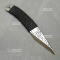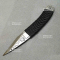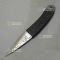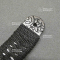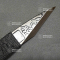ref. : 11882

22,00

Available quantity : 8Order

###### Description

Length 200 mm, width 30 mm. Measurements of the blade: 65 mm. Made of laminated steel  this traditional tool is for grafting wounds. Taking the curved handle in your hand, use your thumb to push the blade upwards against the wound. A perfectly clean cut ensures proper healing of the branch.
Don't forget to view other relevant products:

#blade 4.5 #grafting 3.8 #handle 3.6 #japanese 2.8 #bonsai 2.6 #tools 2.5 #right 2.5 #your 2.4 #measurements 2.2 #traditional 2.1

###### Technical description
 I would like to...

Formule
(( ROUND((CHAR_LENGTH(b.article_nom)-CHAR_LENGTH(REPLACE(b.article_nom, 'blade', '')))/LENGTH('blade')) + ROUND((CHAR_LENGTH(b.article_description)-CHAR_LENGTH(REPLACE(b.article_description, 'blade', '')))/LENGTH('blade')) ) * 4.5) + (( ROUND((CHAR_LENGTH(b.article_nom)-CHAR_LENGTH(REPLACE(b.article_nom, 'grafting', '')))/LENGTH('grafting')) + ROUND((CHAR_LENGTH(b.article_description)-CHAR_LENGTH(REPLACE(b.article_description, 'grafting', '')))/LENGTH('grafting')) ) * 3.8) + (( ROUND((CHAR_LENGTH(b.article_nom)-CHAR_LENGTH(REPLACE(b.article_nom, 'handle', '')))/LENGTH('handle')) + ROUND((CHAR_LENGTH(b.article_description)-CHAR_LENGTH(REPLACE(b.article_description, 'handle', '')))/LENGTH('handle')) ) * 3.6) + (( ROUND((CHAR_LENGTH(b.article_nom)-CHAR_LENGTH(REPLACE(b.article_nom, 'japanese', '')))/LENGTH('japanese')) + ROUND((CHAR_LENGTH(b.article_description)-CHAR_LENGTH(REPLACE(b.article_description, 'japanese', '')))/LENGTH('japanese')) ) * 2.8) + (( ROUND((CHAR_LENGTH(b.article_nom)-CHAR_LENGTH(REPLACE(b.article_nom, 'right', '')))/LENGTH('right')) + ROUND((CHAR_LENGTH(b.article_description)-CHAR_LENGTH(REPLACE(b.article_description, 'right', '')))/LENGTH('right')) ) * 2.5) + (( ROUND((CHAR_LENGTH(b.article_nom)-CHAR_LENGTH(REPLACE(b.article_nom, 'your', '')))/LENGTH('your')) + ROUND((CHAR_LENGTH(b.article_description)-CHAR_LENGTH(REPLACE(b.article_description, 'your', '')))/LENGTH('your')) ) * 2.4) + (( ROUND((CHAR_LENGTH(b.article_nom)-CHAR_LENGTH(REPLACE(b.article_nom, 'measurements', '')))/LENGTH('measurements')) + ROUND((CHAR_LENGTH(b.article_description)-CHAR_LENGTH(REPLACE(b.article_description, 'measurements', '')))/LENGTH('measurements')) ) * 2.2) + (( ROUND((CHAR_LENGTH(b.article_nom)-CHAR_LENGTH(REPLACE(b.article_nom, 'traditional', '')))/LENGTH('traditional')) + ROUND((CHAR_LENGTH(b.article_description)-CHAR_LENGTH(REPLACE(b.article_description, 'traditional', '')))/LENGTH('traditional')) ) * 2.1) + (( ROUND((CHAR_LENGTH(b.article_nom)-CHAR_LENGTH(REPLACE(b.article_nom, 'laminated', '')))/LENGTH('laminated')) + ROUND((CHAR_LENGTH(b.article_description)-CHAR_LENGTH(REPLACE(b.article_description, 'laminated', '')))/LENGTH('laminated')) ) * 1.9) + (( ROUND((CHAR_LENGTH(b.article_nom)-CHAR_LENGTH(REPLACE(b.article_nom, 'perfectly', '')))/LENGTH('perfectly')) + ROUND((CHAR_LENGTH(b.article_description)-CHAR_LENGTH(REPLACE(b.article_description, 'perfectly', '')))/LENGTH('perfectly')) ) * 1.9)

## Secure payment## Delivery

Our logistic partners :04 74 55 23 48
Pépinière MAILLOT-BONSAÏ
Le Bois Frazy
01990 RELEVANT - FRANCE
on appointment